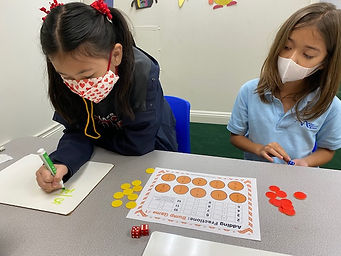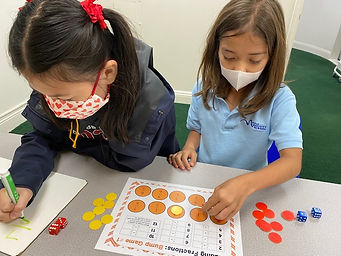Ms. Dawn

Target 1​

Continuation

Number Operation

:

Computation

1:

Understand the arithmetic process used for adding and subtracting fractions with like denominators.

2:

Recognize that only the numerators should be added or subtracted, not the denominators.

3:

Simplify the results of an addition or subtraction problem.

4th

Vocabulary:

Like Denominators, Fraction, Lowest terms, Factors, Numerator, Simplify

Activities:

1. Discussed the rules when you add and subtract fractions with like denominators.

2. Students answered a few equations on their whiteboard to show their understanding.

3. Students played a game called "Fraction Bump." Students rolled two dice and added it up to figure the sum. They needed to match the sum they rolled to the matching problem. Students needed to answer the addition fraction equation etiher on their whiteboard or verbally. If the student got it correct, they would cover it with their color piece. They take turns until someone has used up all of their 10 color pieces. Students could lock in the fraction if they had that answer twice.Home Exploration

Guiding Questions:Absent Students:

Target 2

:

1:

Understand how the concept of multiplication can be applied to fractions.

4th

Vocabulary:

Whole Number, Fraction, Numerator, Denominator, Multiply, One-Digit, Two-Digit

Activities:

1. Discussed the rules of multiplying fractions. Addressing the fact that the whole number turns into a fraction by placing the whole number in the numerator place and one in the denominator place. Then, understanding that it's multiplied straight across.

2. Students were given a set of whole numbers to multiply by fractions. Started off with one-digit whlole numbers and as students started to master those, created two-digit whole numbers for students to multiply.Home Exploration

Guiding Questions:Target 3

:

Vocabulary:

Activities:Home Exploration